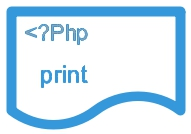# print command in PHP``print " Hello world ";``
Output
``Hello world``
print takes one argument and always returns 1.

## print with Variable

This is without any parentheses ( we can use pint with or without parentheses )
``````\$first="Hello World";
print \$first;``````
Output is here
``Hello World``

## Multiple variables

``````\$first="Hello";
\$second="World";
print   ("\$first");
print "<br>";
print ("\$first \$second");``````
Output is here
``````Hello
Hello World``````

## Multiple Lines

``````print " Line one
Line two
One more line and end ";``````
Output is here
``Line one Line two One more line and end``

## With escaping characters

``print " Welcome to \"plus2net.com\" PHP section ";``
Output is here
``Welcome to "plus2net.com" PHP section``

## Using single quotes

``````\$section = "PHP";
print ' Welcome to \$section of plus2net';``````
Output is here
``Welcome to \$section of plus2net``
We used the variable \$section in above code. It will not print the value of the variable, only name will be printed.

## Using HTML tags

``print " Welcome to <i>plus2net</i> PHP Section " ;``
Output is here
``Welcome to plus2net PHP Section``Subscribe to our YouTube Channel here

## Subscribe

* indicates required
Subscribe to plus2netplus2net.com